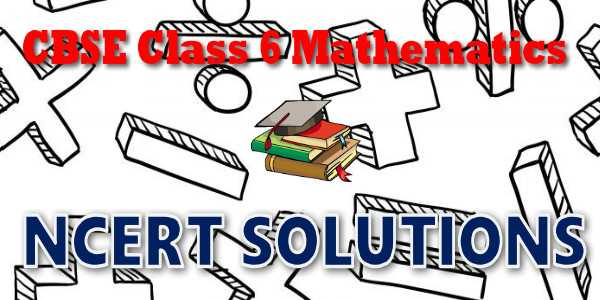# CBSE - Class 06 - Mathematics - Algebra - NCERT Solutions## CBSE, JEE, NEET, NDA

Question Bank, Mock Tests, Exam Papers

NCERT Solutions, Sample Papers, Notes, Videos

## NCERT Solutions for Mathematics Algebra## myCBSEguide App

Complete Guide for CBSE Students

NCERT Solutions, NCERT Exemplars, Revison Notes, Free Videos, CBSE Papers, MCQ Tests & more.

# NCERT solutions for class 6  Mathematics Algebra## NCERT Class 6 Mathematics Chapter-wise Solutions

Maths Part I

• Chapter 1 - Knowing Our Numbers
• Chapter 2 - Whole Numbers
• Chapter 3 - Playing with Numbers
• Chapter 4 - Basic Geometrical Ideas
• Chapter 5 - Understanding Elementary Shapes
• Chapter 6 - Integers
• Chapter 7 - Fractions
• Chapter 8 - Decimals
• Chapter 9 - Data Handling
• Chapter 10 - Mensuration
• Chapter 11 - Algebra
• Chapter 12 - Ratio and Proportion
• Chapter 13 - Symmetry
• Chapter 14 - Practical Geometry

## CBSE class 6th Mathematics have one books. Each book has chapters and topics.

• NCERT Mathematics Book Class 6

Here is the list of topics covered under each chapter of class 6 Mathematics NCERT text book.

• Exercise 1.1
• Exercise 1.2
• Exercise 1.3

• Exercise 2.1
• Exercise 2.2
• Exercise 2.3

• Exercise 3.1
• Exercise 3.2
• Exercise 3.3
• Exercise 3.4
• Exercise 3.5
• Exercise 3.6
• Exercise 3.7

• Exercise 4.1
• Exercise 4.2
• Exercise 4.3
• Exercise 4.4
• Exercise 4.5
• Exercise 4.6

• Exercise 5.1
• Exercise 5.2
• Exercise 5.3
• Exercise 5.4
• Exercise 5.5
• Exercise 5.6
• Exercise 5.7
• Exercise 5.8
• Exercise 5.9

• Exercise 6.1
• Exercise 6.2
• Exercise 6.3

• Exercise 7.1
• Exercise 7.2
• Exercise 7.3
• Exercise 7.4
• Exercise 7.5
• Exercise 7.6

• Exercise 8.1
• Exercise 8.2
• Exercise 8.3
• Exercise 8.4
• Exercise 8.5
• Exercise 8.6

• Exercise 9.1
• Exercise 9.2
• Exercise 9.3
• Exercise 9.4

### 10. Mensuration

• Exercise 10.1
• Exercise 10.2
• Exercise 10.3

### 11. Algebra

• Exercise 11.1
• Exercise 11.2
• Exercise 11.3
• Exercise 11.4
• Exercise 11.5

### 12. Ratio and Proportion

• Exercise 12.1
• Exercise 12.2
• Exercise 12.3

### 13. Symmetry

• Exercise 13.1
• Exercise 13.2
• Exercise 13.3

### 14. Practical Geometry

• Exercise 14.1
• Exercise 14.2
• Exercise 14.3
• Exercise 14.4
• Exercise 14.5
• Exercise 14.6

## NCERT Solutions Chapter 11 Algebra

Our study so far has been with numbers and shapes. We have learnt numbers, operations on numbers and properties of numbers. We applied our knowledge of numbers to various problems in our life. The branch of mathematics in which we studied numbers is arithmetic. We have also learnt about figures in two and three dimensions and their properties. The branch of mathematics in which we studied shapes is geometry. Now we begin the study of another branch of mathematics. It is called algebra. The main feature of the new branch which we are going to study is the use of letters. Use of letters will allow us to write rules and formulas in a general way. By using letters, we can talk about any number and not just a particular number. Secondly, letters may stand for unknown quantities. By learning methods of determining unknowns, we develop powerful tools for solving puzzles and many problems from daily life. Thirdly, since letters stand for numbers, operations can be performed on them as on numbers. This leads to the study of algebraic expressions and their properties. You will find algebra interesting and useful. It is very useful in solving problems. Let us begin our study with simple examples.

## NCERT Solutions for Class 6th Mathematics

NCERT Solutions Class 6 Mathematics PDF (Download) Free from myCBSEguide app and myCBSEguide website. Ncert solution class 6 Mathematics includes text book solutions from  NCERT Solutions for CBSE Class 6 Mathematics have total 14 chapters. Class 6 Mathematics ncert Solutions in pdf for free Download are given in this website. Ncert Mathematics class 6 solutions PDF and Mathematics ncert class 6 PDF solutions with latest modifications and as per the latest CBSE syllabus are only available in myCBSEguide.

## NCERT Solutions for Class 5

NCERT Solutions for Class 4

## NCERT Solutions for Class 3## myCBSEguide

Trusted by 1 Crore+ Students

#### CBSE Test Generator

Create papers in minutes

Print with your name & Logo

3 Lakhs+ Questions

Solutions Included

Based on CBSE Blueprint

Best fit for Schools & Tutors

#### Work from Home

• Work from home with us
• Create questions or review them from home

No software required, no contract to sign. Simply apply as teacher, take eligibility test and start working with us. Required desktop or laptop with internet connection